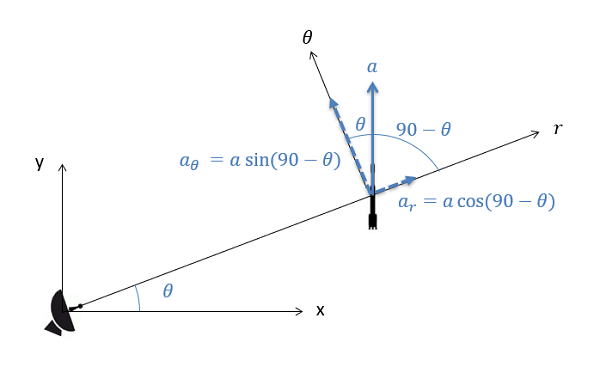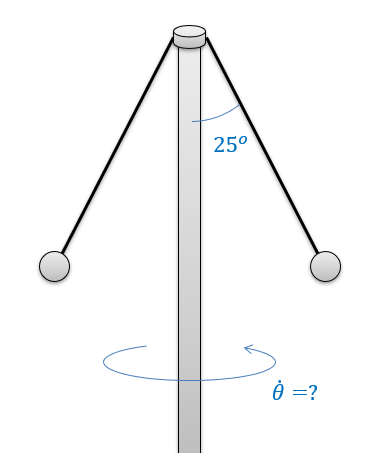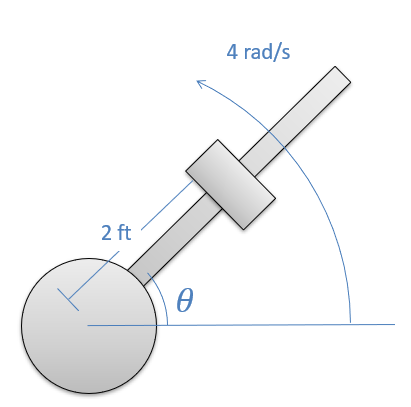﻿ Mechanics Map - The Equations of Motion with Polar Coordinates

# The Equations of Motion with Polar Coordinates

To finish our discussion of the equations of motion in two dimensions, we will examine Newton's Second law as it is applied to the polar coordinate system. In its basic form, Newton's Second Law states that the sum of the forces on a body will be equal to mass of that body times the rate of acceleration. For bodies in motion, we can write this relationship out as the equation of motion.

 $\sum \vec{F}=m*\vec{a}$

Just as we did with with rectangular and normal-tangential coordinates, we will break this single vector equation into two separate scalar equations. This involves identifying the r and theta directions and then using sines and cosines to break the given forces and accelerations down into components in those directions.When working in the polar coordinate system, any given forces or accelerations can be broken down using sines and cosines assuming the angle of the force or acceleration is known relative to the r and theta directions.
 $\sum F_{r}=m*a_{r}$ $\sum F_{\theta}=m*a_{\theta}$

Just as with our other coordinate systems, the equations of motion are often used in conjunction with the kinematics equations, which relate positions, velocities and accelerations as discussed in the previous chapter. In particular, we will often substitute the known values below for the r and theta components for acceleration.

 $a_{r}=\ddot{r}-r\dot{\Theta}^{2}$ $a_{\theta}=2\dot{r}\dot{\Theta}+r\ddot{\Theta}$

Polar coordinates can be used in any kinetics problem, however they work best with problems where there is a stationary body tracking some moving body (such as a radar dish) or there is a particle rotating around some fixed point. These equations will also come back into play when we start examining rigid body kinematics.

## Worked Problems:

### Question 1:

A device consists of two, one-half kilogram masses tethered to a central shaft. The tethers are each .75 meters long and each tether currently makes a 25 degree angle with the central shaft. Assume the central shaft is spinning at a constant rate. What is the rate at which the shaft is spinning? If we want it to spin at exactly 100 rpm, what should the angle of the tethers be?### Question 2:

A catapult design consists of a steel weight on a frictionless rod. The rod spins at a constant rate of 4 radians per second and when theta is 45 degrees from horizontal, the 30 lb weight is released from its position 2 ft from the center of rotation of the shaft. What is the force the shaft exerts on the weight at the instant before and the instant after it is released? What is the acceleration of the weight along the shaft the instant after it is released?